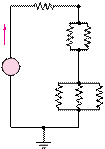### Create an Account

Home / Questions / Cheap resistors are fabricated by depositing a thin layer of carbon onto a nonconducting c...

# Cheap resistors are fabricated by depositing a thin layer of carbon onto a nonconducting cylindrical 252 Use Kirchhoff’s current law and Ohms law to determine the current in each of the

Cheap resistors are fabricated by depositing a thin layer of carbon onto a nonconducting cylindrical

2.52      Use Kirchhoff’s current law and Ohm’s law to determine the current in each of the resistors R4, R5, and R6 in the circuit of Figure P2.52. V= 10 V,

R1 = 20 Q, R2 = 40 Q, R3 = 10 Q, R4 = R5

R6 = 15 Q.R1

I                  R2               R3

 +

+

Jun 19 2020 View more View LessSubscribe To Get Solution